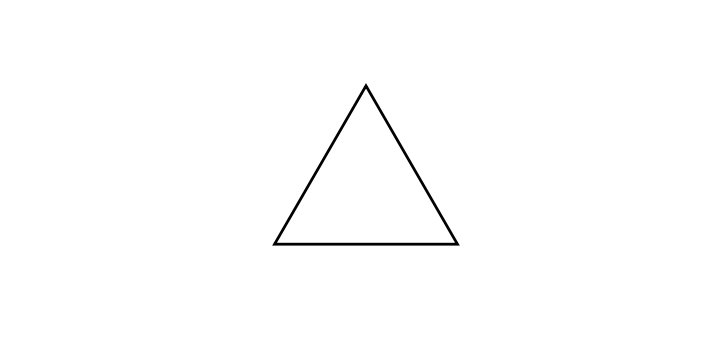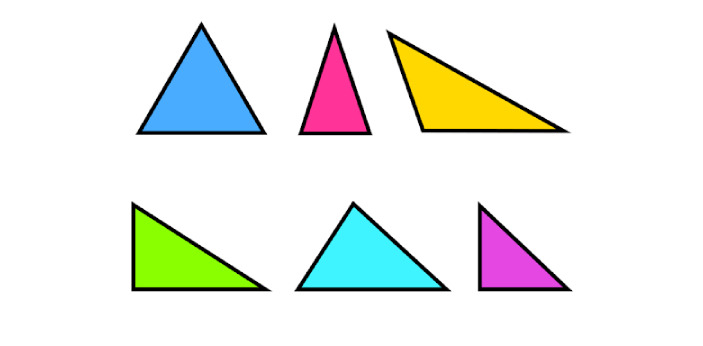How to calculate the area of triangle? - ProProfs Discuss+ Ask Question

# How to calculate the area of triangle?

Asked by Isa , Last updated: Aug 26, 2020

###Request 0FollowShareAnswer AnonymouslyAnswer LaterCopy LinkG. JabezThere are different formulas that you can use if you would like to solve for the area of different shapes. This time, you may need to solve for the area of a triangle.These are the things that you need to do:

• You should first know the formula that you have to use. The formula is going to be A=hb b/2.

• You should know that b stands for the base while hb stands for height.

• Place the required numbers that will correspond to the equation.

Take note that there are also some online calculators that will help you solve for the right answer, but it will still help if you at least know how to solve for the area of a triangle manually.J. Lautner, Product Manager, Utah

There are different ways of calculating the area of a triangle. This is because there are different formulas you can use. However, deciding on the perfect one to use depends solely on the nature of the parameters you are given.1. For the formula we are going to discuss here, you will first need to determine the base of the triangle, as well as the height of the triangle. At times, these two parameters will be given directly in the question.

2. If you want to calculate the area of a triangle using the base and the height of the triangle, you will need this formula i.e., Area= 1/2(bh).

3. For instance, say the base of a triangle is 8 cm, and the height is 6 cm, and you are asked to calculate the area of this triangle, substitute the values for the parameters given.

4. In that case, Area= 1/2(8×6)= 48/2= 24 cm².Search for Google imagesSelect a recommended image
Upload from your computerCancelSearch for Google imagesSelect a recommended image
Upload from your computerCancelSearch for Google imagesSelect a recommended image
Upload from your computerCancel